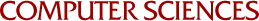## General Convergence Conditions in Nonlinear Programming and a Kuhn-Tucker Algorithm

Hilbert K. Schultz
1971

This paper presents a general definition of algorithmic convergence in mathematical programming and lists conditions which are sufficient for convergence in the sense of the definition. These conditions are also shown to be necessary for convergence in many applications. The definition and conditions are slight modifications of those given by Zangwill [I969 pp. 235 and 244]. Special cases of the assumptions required by Topkis and Veinott  and Polak  for their general algorithms are shown to imply that these general conditions are met, and hence their algorithms converge in the sense of our definition for these cases. The use of the theory is illustrated by proving convergence of the conditional gradient algorithm and a Kuhn-Tucker algorithm for which no prior convergence proof had been given.